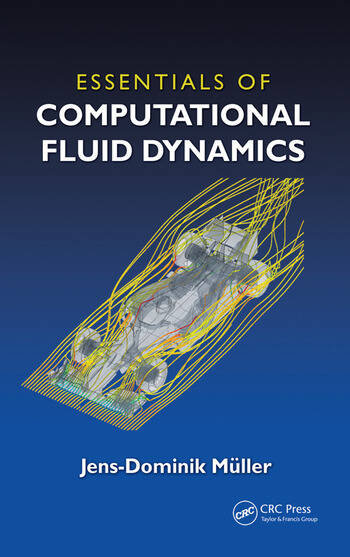Essentials of Computational Fluid Dynamics

1st Edition

Jens-Dominik Mueller

CRC Press
Published November 6, 2015
Textbook - 225 Pages - 103 B/W Illustrations
ISBN 9781482227307 - CAT# K22532

For Instructors Request Inspection Copy

USD\$110.00

FREE Standard Shipping!

Preview

Summary

Covered from the vantage point of a user of a commercial flow package, Essentials of Computational Fluid Dynamics provides the information needed to competently operate a commercial flow solver. This book provides a physical description of fluid flow, outlines the strengths and weaknesses of computational fluid dynamics (CFD), presents the basics of the discretization of the equations, focuses on the understanding of how the flow physics interact with a typical finite-volume discretization, and highlights the approximate nature of CFD. It emphasizes how the physical concepts (mass conservation or momentum balance) are reflected in the CFD solutions while minimizing the required mathematical/numerical background. In addition, it uses cases studies in mechanical/aero and biomedical engineering, includes MATLAB and spreadsheet examples, codes and exercise questions. The book also provides practical demonstrations on core principles and key behaviors and incorporates a wide range of colorful examples of CFD simulations in various fields of engineering.

In addition, this author:

• Introduces basic discretizations, the linear advection equation, and forward, backward and central differences
• Proposes a prototype discretization (first-order upwind) implemented in a spreadsheet/MATLAB example that highlights the diffusive character
• Looks at consistency, truncation error, and order of accuracy
• Analyzes the truncation error of the forward, backward, central differences using simple Taylor analysis
• Demonstrates how the of upwinding produces Artificial Viscosity (AV) and its importance for stability
• Explains how to select boundary conditions based on physical considerations
• Illustrates these concepts in a number of carefully discussed case studies

Essentials of Computational Fluid Dynamics provides a solid introduction to the basic principles of practical CFD and serves as a resource for students in mechanical or aerospace engineering taking a first CFD course as well as practicing professionals needing a brief, accessible introduction to CFD.

Instructors

We provide complimentary e-inspection copies of primary textbooks to instructors considering our books for course adoption.